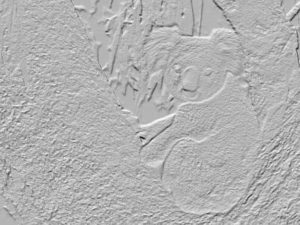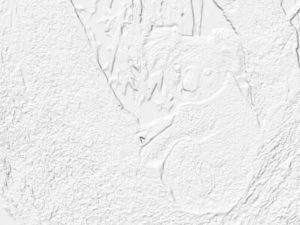# Python – shade() function in Wand

• Last Updated : 18 Oct, 2021

shade() function generates a 3d kind of image or creates a 3d effect by simulating a light from an elevated angle. azimuth parameter is used to control the X and Y angle and elevation parameter is used to control the z angle of the image. We can also get final image in grayscale by putting gray parameter as true.

Syntax :

`wand.image.shade(gray, azimuth, elevation);`

Parameters :

Source Image:Example 1:

## Python3

 `# import Image from wand.image module``from` `wand.image ``import` `Image` `# Read image using Image function``with Image(filename ``=``"koala.jpeg"``) as img:` `    ``# generating shaded image using shade() function.``    ``img.shade(gray ``=` `True``,``              ``azimuth ``=` `286.0``,``              ``elevation ``=` `45.0``)` `    ``img.save(filename ``=``"shadekoala.jpeg"``)`

Output:Example 2: setting gray as False, increasing azimuth and elevation value.

## Python3

 `# import Image from wand.image module` `from` `wand.image ``import` `Image` `with Image(filename ``=``"koala.jpeg"``) as img:``    ``# generating shaded image using shade() function.``    ``img.shade(gray ``=` `True``,``              ``azimuth ``=` `298.0``,``              ``elevation ``=` `70.0``)` `    ``img.save(filename ``=``"shadekoala_2.jpeg"``)`

Output:My Personal Notes arrow_drop_up